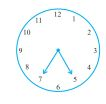Q

# What does the angle made by the hour hand of the clock look like when it moves from 5 to 7. Is the angle moved more than 1 right angle?

What does the angle made by the hour hand of the clock look like when it moves from 5 to 7. Is the angle moved more than 1                   right angle?Views

No, the angle is not more than than 1 right angle.

For each hour, angle made =

Therefore, when the hour hand moves from 5 to 7, the angle made =

Exams
Articles
Questions